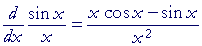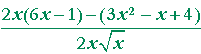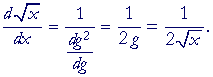An Approach

to

C A L C U L U S

8

# DERIVATIVES

The quotient rule

The quotient rule

The following is called the quotient rule:"The derivative of the quotient of two functions is equal to

the denominator times the derivative of the numerator
minus the numerator times the derivative of the denominator
all divided by the square of the denominator."

For example, accepting for the moment that the derivative of sin x is cos x (Lesson 12):Problem 1.   Calculate the derivative of x2  sin x .

To see the answer, pass your mouse over the colored area.
To cover the answer again, click "Refresh" ("Reload").
Do the problem yourself first!

 sin x· 2x − x2 cos x         sin2x
 Problem 2.   Use the chain rule to calculate the derivative of sin2x  x3 .
 x3· 2 sin x cos x − sin2x· 3x2                 x6 = x2 sin x(2x cos x − 3 sin x)                x6 = sin x(2x cos x − 3 sin x)               x4
 Problem 3.   Calculate the derivative of x2 − 5x − 6    2x + 1 .
 (2x + 1)(2x − 5) − (x2 − 5x − 6)· 2                  (2x + 1)2 = 4x2 − 8x − 5 − 2x2 + 10x + 12                  (2x + 1)2 = 2x2 + 2x + 7   (2x + 1)2
 Problem 4.   Calculate the derivative of 3x2 − x + 4.

See the Example, Lesson 6, and Lesson 22 of Algebra.===Proof of the quotient rule

 THEOREM.Proof.   Since g = g(x), then

 d dx 1g = d dg 1g · dg dx =  − 1g2 g'

according to the chain rule, and Problem 4 of Lesson 5.

Therefore, according to the product rule (Lesson 6),This is the quotient rule, which we wanted to prove.

Implicit differentiation

Consider the following:

x2 + y2 = r2

This is the equation of a circle with radius r. (Lesson 17 of Precalculus.)

 Let us calculate dydx . To do that, we could solve for y and then take the

derivative.  But rather than do that, we will take the derivative of each term.  As for y2, we consider it implicitly a function of x, and therefore

 we may apply the chain rule to it.  Then we will solve for dydx .
 d dx x2 + d dx y2 = ddx r2
 2x + 2y dy dx = 0 dy dx = − xy .

This is called implicit differentiation.  We treat y as a function of x and apply the chain rule.  The derivative that results generally contains both x and y.

Problem 5.   15y + 5y3 + 3y5 = 5x3.  Calculate y'.

 15y' + 15y2y' + 15y4y' = 15x2 y'(1 + y2 + y4) = x2 y' = x2      1 + y2 + y4
 Problem 6.Calculate y'.= 0=y' =Problem 7.

a)  In this circle,

x2 + y2 = 25,

a)   what is the y-coördinate when x = −3?

y = 4 or −4. For,

(−3)2 + (±4)2 = 52

b)  What is the slope of the tangent to the circle at (−3, 4)?

 34 .  For, the derivative is − xy .

c)  What is the slope of the tangent to the circle at (−3, −4)?

 − 34

Problem 8.   In the first quadrant, what is the slope of the tangent to this circle,

(x − 1)2 + (y + 2)2 = 169,

when x = 6?

[Hint:  52 + 122 = 132 is a Pythagorean triple.]

In the first quadrant, when x = 6, y = 10.

(6 − 1)2 + (10 + 2)2 = 132.

 y' = − x − 1y + 2 .  Therefore the slope is − 6 − 110 + 2 =  − 5 12

Problem 9.   Calculate the slope of the tangent to this curve at (2, −1):

x3 − 3xy2 + y3 = 1

 3x2 − (3x· 2y y' + y2· 3) + 3y2 y' = 0 according to the product rule. 3x2 − 6xy y' − 3y2 + 3y2 y' = 0 x2 − 2xy y' − y2 + y2 y' = 0 y'(y2 − 2xy) = y2 − x2 y' = y2 − x2 y2 − 2xy Therefore, at (2, −1): y' = (−1)2 − 22    (−1)2 − 2· 2· −1 = −3  5 = − 35

The derivative of an inverse function

When we have a function  y = f(x) -- for example

y = x2

-- then we can often solve for x.  In this case,On exchanging the variables, we haveis called the inverse function of  y = x2.

Let us write

 f(x) = x2 g(x) =And let us call f the direct function and g the inverse function.  The formal relationship between f and g is the following:

f( g(x)) = g( f(x)) = x.

(Topic 19 of Precalculus.)

Here are other pairs of direct and inverse functions:

 f(x) = sin x g(x) = arcsin x f(x) = ax g(x) = logax f(x) = x3 g(x) =Now, when we know the derivative of the direct function f, then from it we can determine the derivative of g.

Thus, let g(x) be the inverse of f(x).  Then

f(g(x)) = x.

Now take the derivative with respect to x:This implies the following:

Theorem.   If g(x) is the inverse of f(x), then"The derivative of an inverse function is equal to

the reciprocal of the derivative of the direct function

when its argument is the inverse function."

 Example.   Let f(x) = x2,  andThen  f( g) = g2.

Therefore,Next Lesson:  Instantaneous velocity and rates of change

Please make a donation to keep TheMathPage online.
Even \$1 will help.